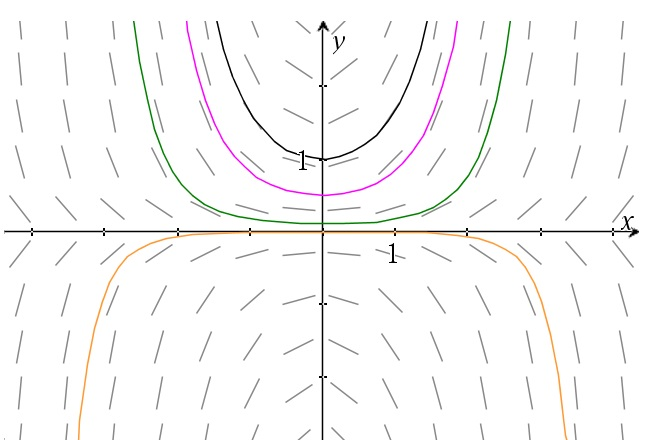# Separable differential equations

Up a level : Differential Equations
Previous page : A few examples from the kinematics
Next page : Separable differential equations - A few illustrating examplesSay we have an equation like$\frac{{dy}}{{dx}} = xy$

This equation tells us what the slope of a solution will be at any given point. If we for example are at (4, 2) then the slope would be 8. A graph of the slopes are given below.Such a graph is called a slope field.

Since each point has one and only one slope, a solution through it would correspond to one and only one curve.  But how to find the solutions? We will do a little trick here and separate the two parts of the differential to get$\frac{{dy}}{y} = xdx$

Next we integrate both sides to get$\int {\frac{{dy}}{y}} = \int {xdx}$

or$\ln |y| = \frac{{{x^2}}}{2} + {C_1}$

We will get a constant of integration from each integral, but they can be combined to one. The above gives us$|y| = {e^{{x^2}/2 + {C_1}}} = {C_2}{e^{{x^2}/2}}$

But since the sign can be absorbed by the new constant we finally get$y = C{e^{{x^2}/2}}$

A graph of a few of these solutions would look something like this.To check that this really is the solution we can differentiate what we have to get$y' = C{e^{{x^2}/2}}x = xy$

But would this trick work in general?

Say we have an equation of the form$\frac{{dy}}{{dx}} = \frac{{f(x)}}{{g(y)}}$

From this we get$g(y)dy = f(x)dx$

or$\int {g(y)dy} = \int {f(x)dx}$

and thus$G(y) = F(x) + C$

as a solution.  Differentiating this with respect to x gives us$g(y)y' = f(x)$

using the chain rule. This gives$y' = \frac{{dy}}{{dx}} = \frac{{f(x)}}{{g(y)}}$

I.e. what we started with.  We can thus solve equations of the form$\frac{{dy}}{{dx}} = \frac{{f(x)}}{{g(y)}}$

by separating the equation to an y and x part.  An equation of the form$\frac{{dy}}{{dx}} = f(x)g(y)$

can be separated too and the proof lies in the fact that multiplying with something is to divide by its reciprocal, so the above can be rewritten in the former form.Up a level : Differential Equations
Previous page : A few examples from the kinematics
Next page : Separable differential equations - A few illustrating examplesLast modified: Mar 13, 2019 @ 20:19